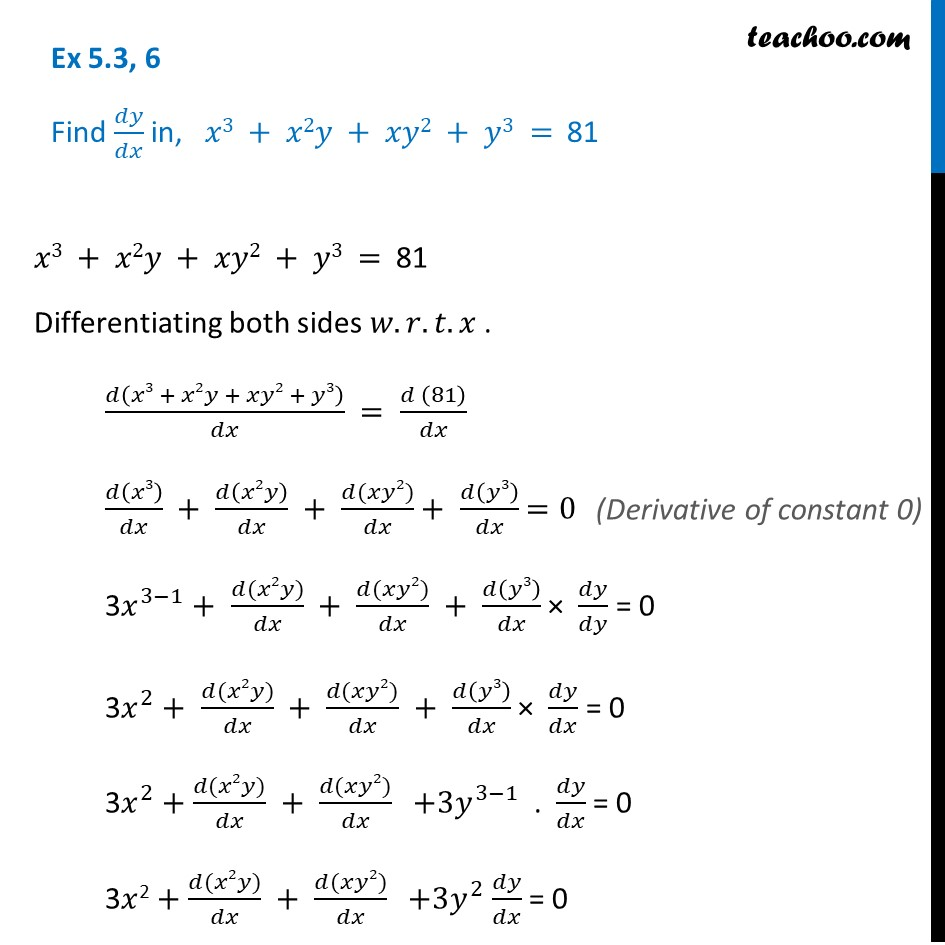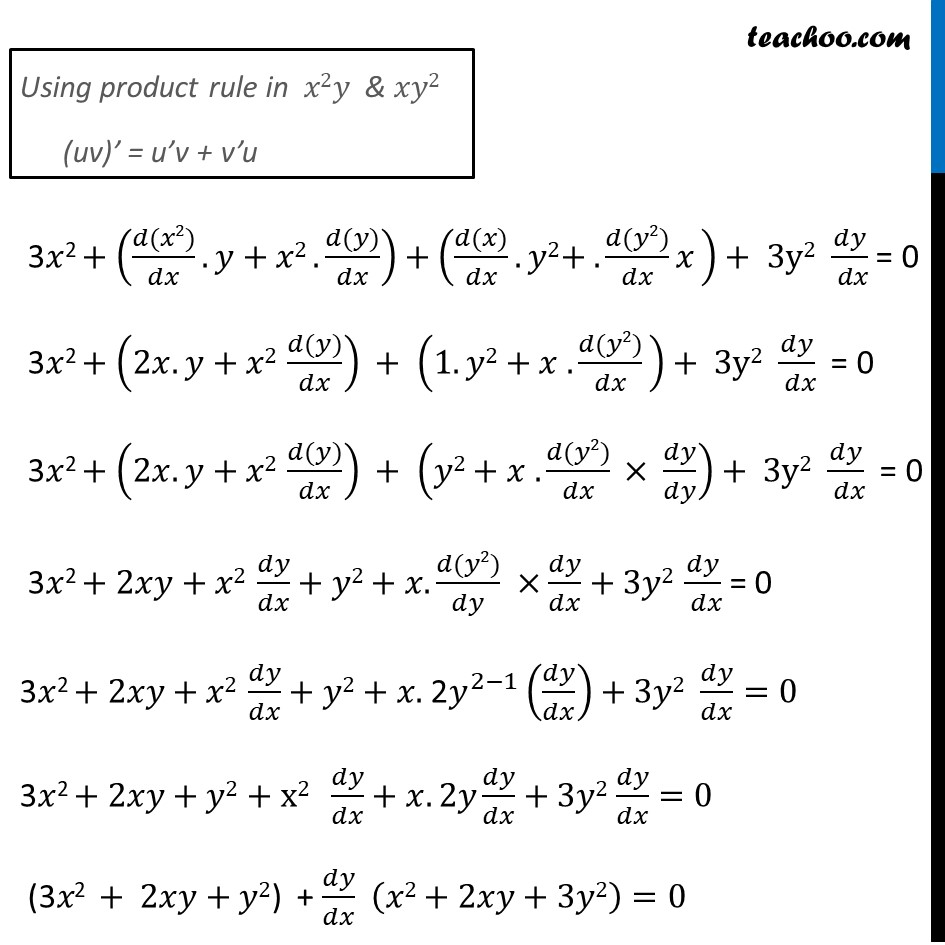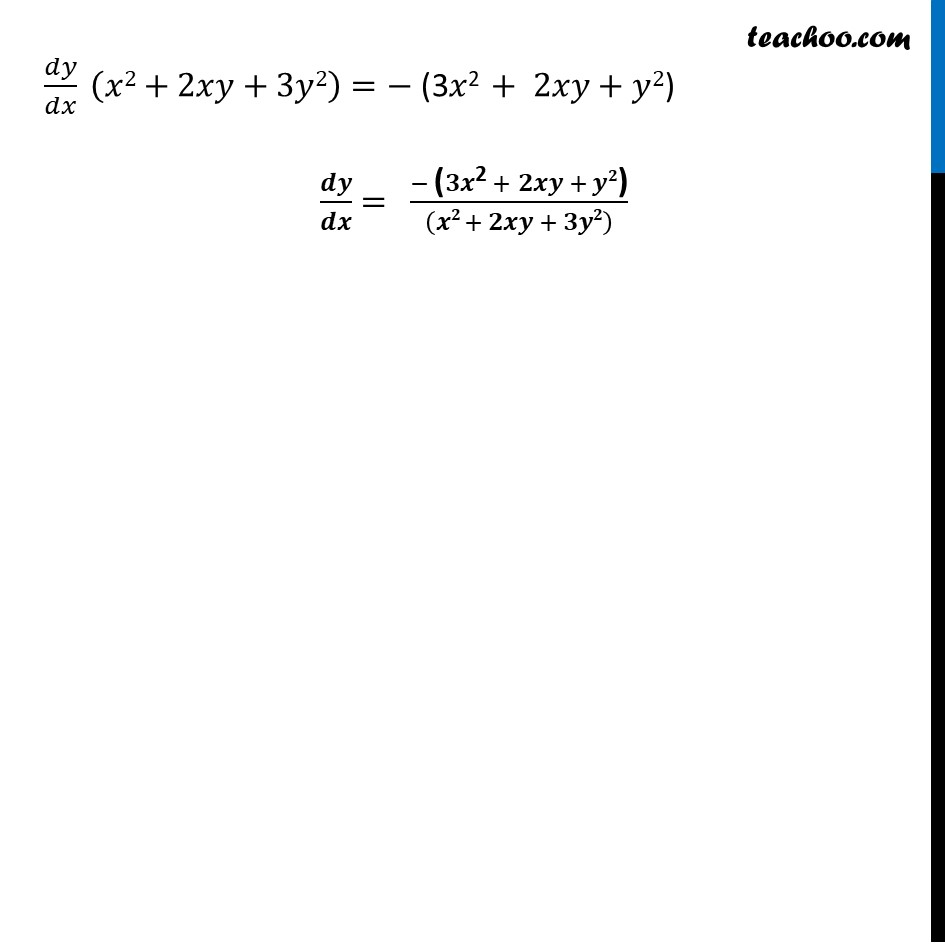Finding derivative of Implicit functions

Chapter 5 Class 12 Continuity and Differentiability
Concept wiseIntroducing your new favourite teacher - Teachoo Black, at only ₹83 per month

### Transcript

Ex 5.3, 6 Find 𝑑𝑦/𝑑𝑥 in, 𝑥3 + 𝑥2𝑦 + 𝑥𝑦2 + 𝑦3 = 81 𝑥3 + 𝑥2𝑦 + 𝑥𝑦2 + 𝑦3 = 81 Differentiating both sides 𝑤.𝑟.𝑡.𝑥 . 𝑑(𝑥3 + 𝑥2𝑦 + 𝑥𝑦2 + 𝑦3)/𝑑𝑥 = (𝑑 (81))/𝑑𝑥 𝑑(𝑥3)/𝑑𝑥 + 𝑑(𝑥2𝑦)/𝑑𝑥 + (𝑑(𝑥𝑦2))/𝑑𝑥 + 𝑑(𝑦3)/𝑑𝑥 =0 3𝑥^(3−1) + (𝑑(𝑥2𝑦))/𝑑𝑥 + (𝑑(𝑥𝑦2))/𝑑𝑥 + 𝑑(𝑦3)/𝑑𝑥 × 𝑑𝑦/𝑑𝑦 = 0 3𝑥^2 + (𝑑(𝑥2𝑦))/𝑑𝑥 + (𝑑(𝑥𝑦2))/𝑑𝑥 + 𝑑(𝑦3)/𝑑𝑥 × 𝑑𝑦/𝑑𝑥 = 0 3𝑥^2+ (𝑑(𝑥2𝑦))/𝑑𝑥 + (𝑑(𝑥𝑦2))/𝑑𝑥 +3𝑦^(3−1) . 𝑑𝑦/𝑑𝑥 = 0 3𝑥2 + (𝑑(𝑥2𝑦))/𝑑𝑥 + (𝑑(𝑥𝑦2))/𝑑𝑥 +3𝑦^2 𝑑𝑦/𝑑𝑥 = 0 Using product rule in 𝑥2𝑦 & 𝑥𝑦2 (uv)’ = u’v + v’u 3𝑥2 + ((𝑑(𝑥2))/𝑑𝑥.𝑦+𝑥2 .(𝑑(𝑦))/𝑑𝑥)+((𝑑(𝑥))/𝑑𝑥.𝑦2+ .(𝑑(𝑦2))/𝑑𝑥 𝑥 )+ 3y2 𝑑𝑦/( 𝑑𝑥) = 0 3𝑥2 + (2𝑥.𝑦+𝑥2 (𝑑(𝑦))/𝑑𝑥) + (1.𝑦2+𝑥 .(𝑑(𝑦2))/𝑑𝑥 )+ 3y2 𝑑𝑦/( 𝑑𝑥) = 0 3𝑥2 + (2𝑥.𝑦+𝑥2 (𝑑(𝑦))/𝑑𝑥) + (𝑦2+𝑥 .(𝑑(𝑦2))/𝑑𝑥 × 𝑑𝑦/𝑑𝑦)+ 3y2 𝑑𝑦/( 𝑑𝑥) = 0 3𝑥2 + 2𝑥𝑦+𝑥2 𝑑𝑦/𝑑𝑥+𝑦2+𝑥.(𝑑(𝑦2))/𝑑𝑦 ×𝑑𝑦/𝑑𝑥+3𝑦2 𝑑𝑦/( 𝑑𝑥) = 0 3𝑥2 + 2𝑥𝑦+𝑥2 𝑑𝑦/𝑑𝑥+𝑦2 + 𝑥. 2𝑦^(2−1) (𝑑𝑦/𝑑𝑥)+3𝑦2 𝑑𝑦/𝑑𝑥=0 3𝑥2 + 2𝑥𝑦+𝑦2 + x2 𝑑𝑦/𝑑𝑥+𝑥.2𝑦 𝑑𝑦/𝑑𝑥+3𝑦2 𝑑𝑦/𝑑𝑥=0 "(3" 𝑥"2 "+" " 2𝑥𝑦+𝑦2")" + 𝑑𝑦/𝑑𝑥 (𝑥2+2𝑥𝑦+3𝑦2)=0 𝑑𝑦/𝑑𝑥 (𝑥2+2𝑥𝑦+3𝑦2)=− "(3" 𝑥"2 "+" " 2𝑥𝑦+𝑦2")" 𝒅𝒚/𝒅𝒙= (− "(" 𝟑𝒙"2 " +" " 𝟐𝒙𝒚 + 𝒚𝟐")" )/((𝒙𝟐 + 𝟐𝒙𝒚 + 𝟑𝒚𝟐) )